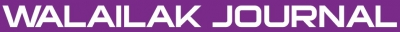### A Legendre Computational Matrix Method for Solving High-Order Fractional Differential Equations

Mohamed Meabed KHADER, Ahmed Saied HENDY

#### Abstract

In this paper, a matrix method for the approximate solution of high order fractional differential equations (FDEs) in terms of a truncated Legendre series is presented. The FDEs and its initial or boundary conditions are transformed to matrix equations, which correspond to a system of algebraic equations with unknown Legendre coefficients. The solution of this system yields the Legendre coefficients of the solution formula. Several numerical examples, such as Cauchy and Bagley-Torvik fractional differential equations, are provided to confirm the accuracy and the effectiveness of the proposed method.

doi:10.14456/WJST.2014.45

#### Keywords

Ordinary fractional differential equations, shifted Legendre polynomials, Caputo derivatives, computational matrix method, cauchy equations, Bagley-Torvik equations

PDF

#### References

S Das. Functional Fractional Calculus for System Identification and Controls. Springer, New York, 2008.

D Funaro. Polynomial Approximation of Differential Equations. Springer Verlag, New York, 1992.

MM Khader. Introducing an efficient modification of the variational iteration method by using Chebyshev polynomials. Appl. Appl. Math.2012; 7, 283-99.

MM Khader and AS Hendy. The approximate and exact solutions of the fractional-order delay differential equations using Legendre pseudospectral method. Int. J. Pure Appl. Math. 2012; 74, 287-97.

MM Khader and AS Hendy. A numerical technique for solving fractional variational problems. Math. Meth. Appl. Sci. 2013; 36, 1281-9.

MM Khader, TS EL Danaf and AS Hendy. Efficient spectral collocation method for solving multi-term fractional differential equations based on the generalized Laguerre polynomials. J. Fractional Calc. Appl. 2012; 3, 1-14.

S Kumar, H Kocak and A Yildirim. A fractional model of Gas dynamics equations and its analytical approximate solution using Laplace transform. Z. Naturforsch 2012; 67a, 389-96.

NH Sweilam and MM Khader. A Chebyshev pseudo-spectral method for solving fractional integro-differential equations. ANZIAM J. 2010; 51, 464-75.

NH Sweilam, MM Khader and A Adel. On the stability analysis of weighted average finite difference methods for fractional wave equation. Fractional Differ. Calc. 2012; 2, 17-29.

NH Sweilam, MM Khader and AMS Mahdy. Crank-Nicolson finite difference method for solving time-fractional diffusion equation. J. Fract. Calcul. Appl. 2012; 2, 1-9.

NH Sweilam, MM Khader and AMS Mahdy. Numerical studies for fractional-order Logistic differential equation with two different delays. J. Appl. Math. 2012; 2012, 1-14.

NH Sweilam, MM Khader and AMS Mahdy. Numerical studies for solving fractional-order Logistic equation. Int. J. Pure Appl. Math. 2012; 78, 1199-210.

I Timucin. Chebyshev collocation method for solving linear differential equations. Math. Comput. Appl. 2004; 9, 107-15.

M Gulsu, Y Ozturk and M Sezer. On the solution of the Abel equation of the second kind by the shifted Chebyshev polynomials. Appl. Math. Comput. 2011; 217, 4827-33.

Y Li and W Zhao. Haar wavelet operational matrix of fractional order integration and its applications in solving the fractional order differential equations. Appl. Math. Comput. 2010; 216, 2276-85.

MM Khader. On the numerical solutions for the fractional diffusion equation. Commun. Nonlinear Sci. Numer. Simulat. 2011; 16, 2535-42.

MM Khader. Numerical solution of nonlinear multi-order fractional differential equations by implementation of the operational matrix of fractional derivative. Stud. Nonlinear Sci. 2011; 2, 5-12.

EH Doha, AH Bhrawy and SS Ezz-Eldien. Efficient Chebyshev spectral methods for solving multi-term fractional orders differential equations. Appl. Math. Model. 2011; 35, 5662-72.

J Dascioglu and H Yaslan. The solution of high-order nonlinear ordinary differential equations by Chebyshev series. Appl. Math. Comput. 2011; 217, 5658-66.

AH Bhrawy, AS Alofi and SS Ezz-Eldien. A quadrature Tau method for fractional differential equations with variable coefficients. Appl. Math. Lett. 2011; 24, 2146-52.

AH Bhrawy and AS Alofi. A Jacobi-Gauss collocation method for solving nonlinear Lane-Emden type equations. Commun. Nonlinear Sci. Numer. Simulat. 2012; 17, 62-70.

C Canuto, MY Hussaini, A Quarteroni and TA Zang. Spectral Methods. Springer-Verlag, New York, 2006.

EH Doha, AH Bhrawy and RM Hafez. On shifted Jacobi spectral method for high-order multi-point boundary value problems. Commun. Nonlinear Sci. Numer. Simulat. 2012; 17, 3802-10.

BY Guo and JP Yan. Legendre-Gauss collocation method for initial value problems of second order ordinary differential equations. Appl. Numer. Math. 2009; 59, 1386-408.

A Saadatmandi and M Dehghan. The use of Sinc-collocation method for solving multi-point boundary value problems. Commun. Nonlinear Sci. Numer. Simulat. 2012; 17, 593-601.

AH Bhrawy and M Alghamdi. A shifted Jacobi-Gauss-Lobatto collocation method for solving nonlinear fractional Langevin equation. Bound. Value Probl. 2012; 2012, Article ID 62.

EH Doha, AH Bhrawy and SS Ezz-Eldien. A new Jacobi operational matrix: an application for solving fractional differential equations. Appl. Math. Model. 2012; 36, 4931-43.

EH Doha, AH Bhrawy and SS Ezz-Eldien. A Chebyshev spectral method based on operational matrix for initial and boundary value problems of fractional order. Comput. Math. Appl. 2011; 62, 2364-73.

A Saadatmandi and M Dehghan. A new operational matrix for solving fractional-order differential equations. Comput. Math. Appl. 2010; 59, 1326-36.

AH Bhrawy and M Alshomrani. A shifted Legendre spectral method for fractional-order multi-point boundary value problems. Adv. Differ. Equat. 2012; 2012, Article ID 8.

S Yuzbasi. Numerical solution of the Bagley-Torvik equation by the Bessel collocation method. Math. Meth. Appl. Sci. 2013; 36, 300-12.

AH Bhrawy and AS Alofi. The operational matrix of fractional integration for shifted Chebyshev polynomials. Appl. Math. Lett. 2013; 26, 25-31.

Y Li and N Sun. Numerical solution of fractional differential equations using the generalized block pulse operational matrix. Comput. Math. Appl. 2011; 62, 1046-54.

A Deng and L Ma. Existence and uniqueness of initial value problems for non-linear fractional differential equations. Appl. Math. Lett. 2010; 23, 676-80.

M Abramowitz and IA Stegun. Handbook of Mathematical Functions. Dover, New York, 1964.

S Samko, A Kilbas and O Marichev. Fractional Integrals and Derivatives: Theory and Applications. Gordon and Breach, London, 1993.

### Refbacks

• There are currently no refbacks.Online ISSN: 2228-835X

http://wjst.wu.ac.th

Last updated: 20 June 2019